# Price Elasticity

Describing the relationship between price and quantity

## What is Price Elasticity?

Price elasticity refers to how the quantity demanded or supplied of a good changes when its price changes. In other words, it measures how much people react to a change in the price of an item.

Price elasticity of demand refers to how changes to price affect the quantity demanded of a good. Conversely, price elasticity of supply refers to how changes in price affect the quantity supplied of a good.### Price Elasticity of Demand

There are three main types of price elasticity of demand: elastic, unit elastic, and inelastic. Before delving deeper into the subject, a sound understanding of the laws of supply and demand is recommended.

To calculate the Price Elasticity of Demand (PED), we use the following equation: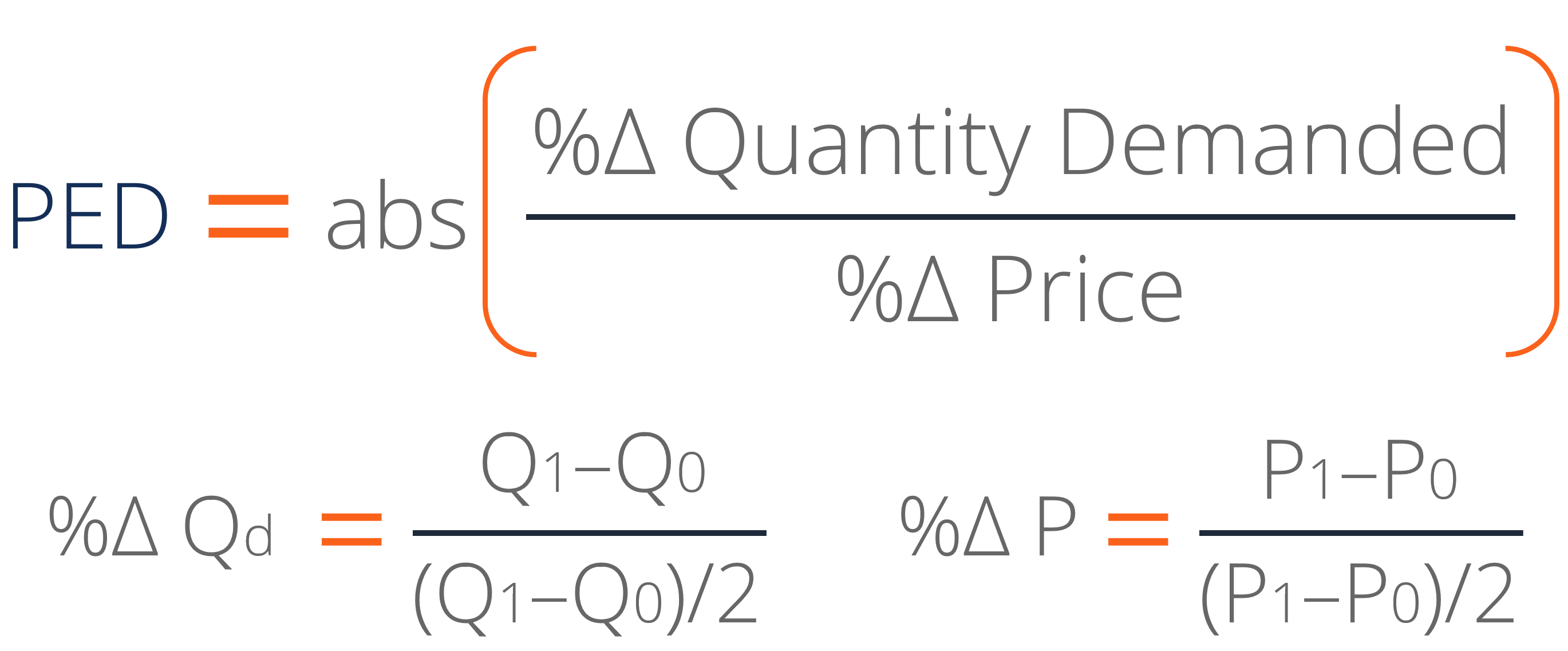Where:

% Change in Quantity Demanded (Qd) = (New Quantity – Old Quantity)/Average Quantity

% Change in Price (P) = (New Price – Old Price)/Average Price

PED is always provided as an absolute value, or positive value, as we are interested in its magnitude.

### Midpoint Method for Elasticity

Some economics resources will instead calculate price elasticity using the following formulas:

% Change in Quantity Demanded (Qd) = (New Quantity – Old Quantity)/Old Quantity

% Change in Price (P) = (New Price – Old Price)/Old Price

Notice that the denominators for both of these are the old quantity and price as opposed to the average price and quantity that was shown above. Using this formula is not ideal because the direction of the change in price or quantity can affect the number calculated for price elasticity.

Here is an example to illustrate this. The cost of a pair of pants drops from \$30 to \$20 and the quantity demanded goes from 100 to 150 pairs of pants. The price elasticity of demand calculation for this would be as follows: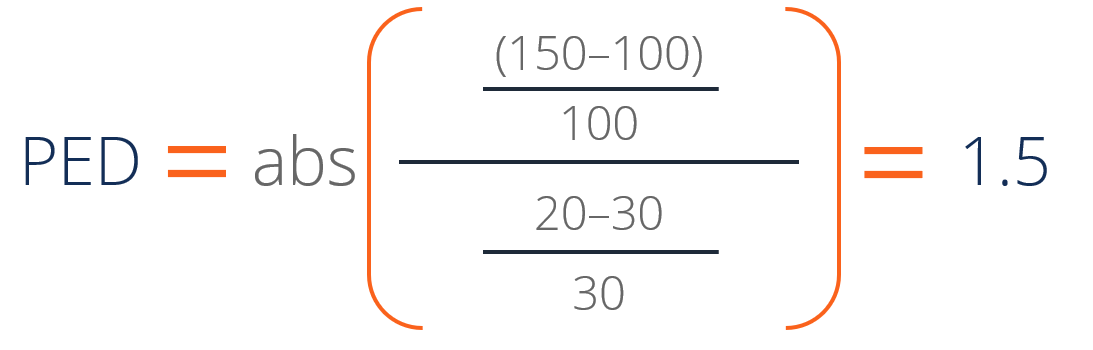However, if we flip this example and the pair of pants is increasing in price, we get this calculation instead: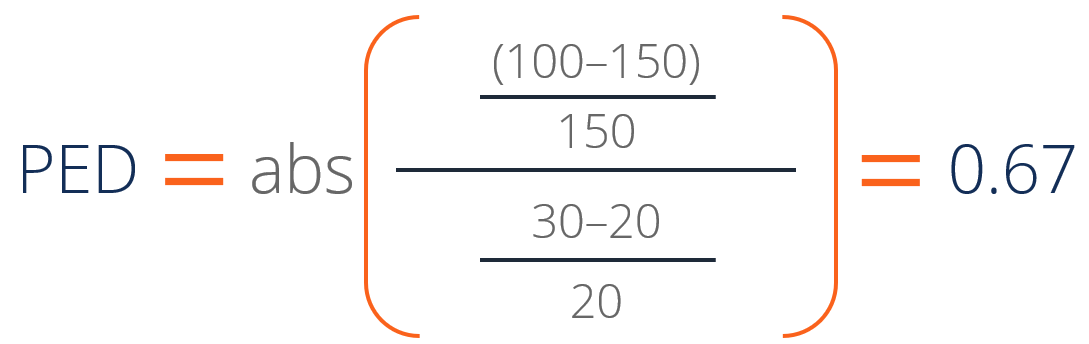In this example, the numbers mentioned are the same, and the change is the exact same. The only difference is that the direction of the changes is different, causing different price elasticities of demand. To solve this, the formula that we use above employs the midpoint method for elasticity.

The midpoint method uses the average quantity and price as the denominators for the percentage change formulas as follows:

% Change in Quantity Demanded (Qd) = (New Quantity – Old Quantity)/Average Quantity

% Change in Price (P) = (New Price – Old Price)/Average Price

This solves the problem of the differing elasticities, as we can see using the following calculations for the previous example: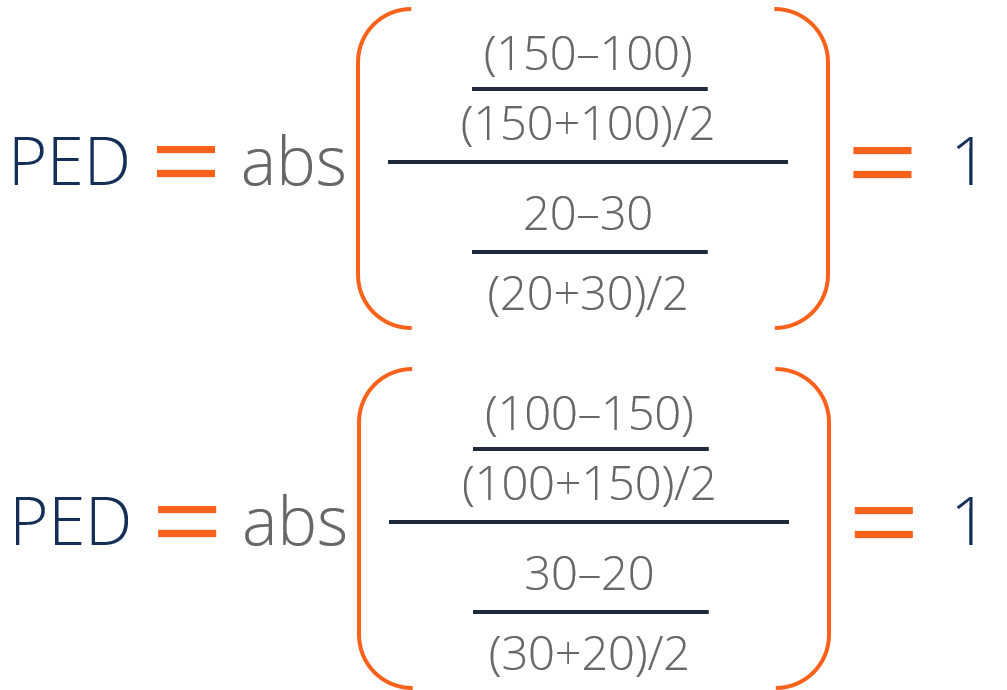### Elastic Demand

Elastic demand occurs when changes in price cause a disproportionately large change in quantity demanded. For example, a good with elastic demand might see its price increase by 10%, but demand drop by 30% as a result. Goods that experience this kind of demand are labeled as “price sensitive,” and are typically non-essential goods that have many substitutes (such as restaurant meals, fashion items, etc.).

A good is considered to be “elastic” when its PED is greater than 1. For example, if the quantity demanded of a handbag drops from 300 to 200 when a price increases from \$500 to \$550, the handbag’s PED would be: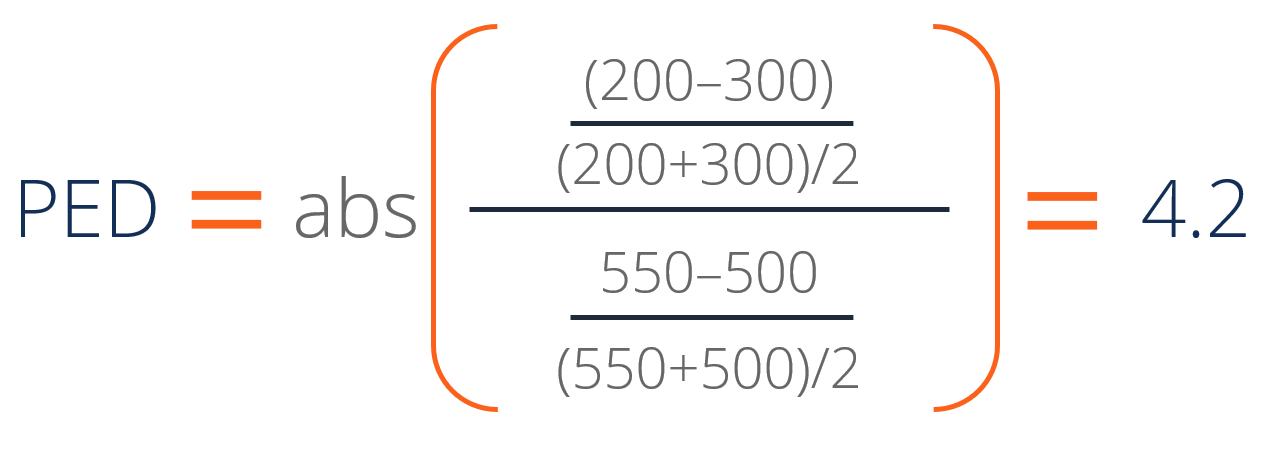The PED of the good is 4.2, which is considered to be elastic.

A good with perfectly elastic demand would have a PED of infinity, where even minuscule changes in price would cause an infinitesimally large change in demand.

### Inelastic Demand

Inelastic demand occurs when changes in price cause a disproportionately small change in quantity demanded. For example, a good with inelastic demand might see its price increase by 30%, but demand drop by only 10% as a result. Goods that experience this kind of demand are labeled as being “price insensitive,” and are typically essential goods that consumers have no substitutes for (such as water, medication, cigarettes, etc.).

A good is considered to be “inelastic” when its PED is less than 1. For example, if the quantity demanded of a cancer treatment drug drops from 900 to 700 when a price increases from \$500 to \$900, the drug’s PED would be: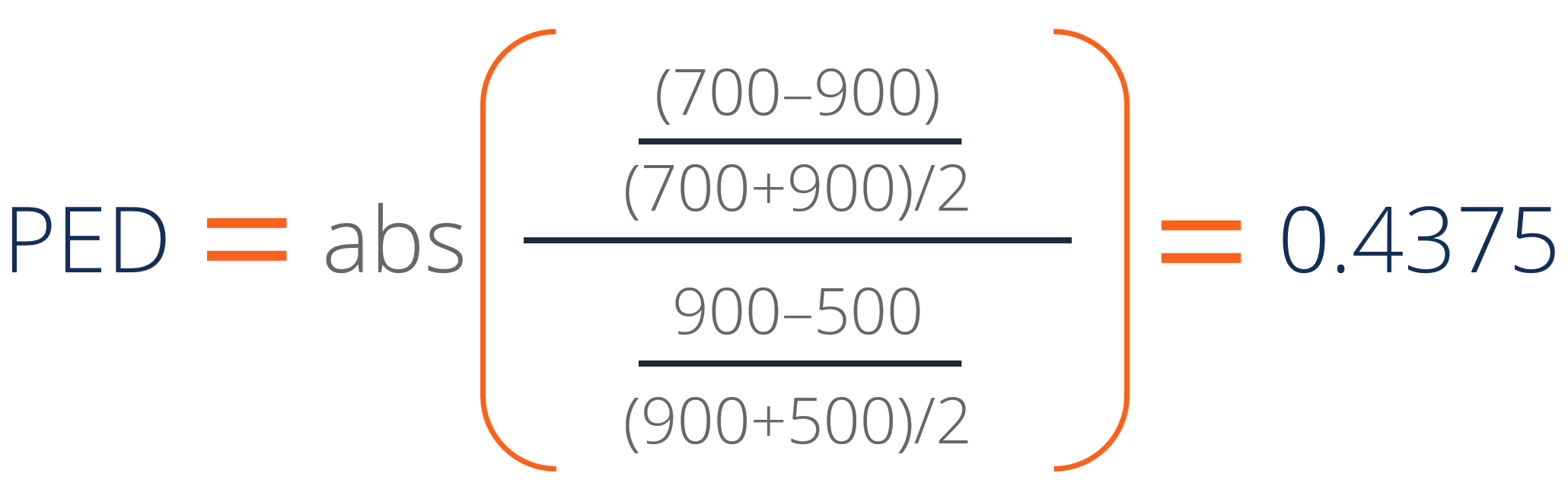The PED of the good is 0.4375, which is considered to be inelastic.

A good with perfectly inelastic demand would have a PED of 0, where even huge changes in price would cause no change in demand.

### Unit Elastic Demand

Unit elastic demand occurs when changes in price cause an equally proportional change in quantity demanded. For example, a good with inelastic unit elastic demand might see its price increase by 30%, and demand would also drop by 30%. Such goods are more difficult to find in markets today, and unit elastic demand is more of a theoretical economics concept. Nonetheless, a good with unit elastic demand could exist.

A good is considered to be “unit elastic” when its PED is equal to 1. For example, if the quantity demanded of a good drops from 1,000 to 900 when a price increases from \$90 to \$100, the good’s PED would be: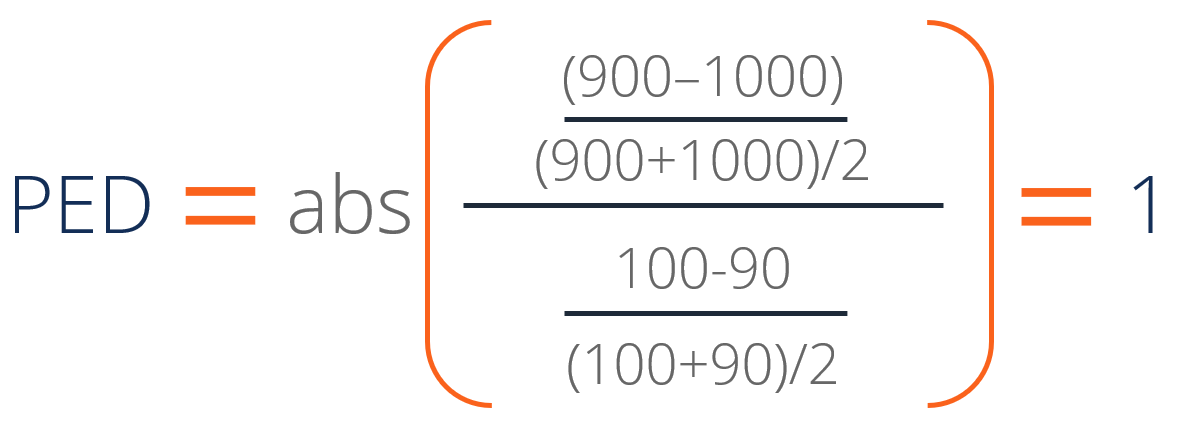The PED of the good is 1which is considered to be unit elastic.

### Price Elasticity of Supply

Price elasticity of supply (PES) works in the same way that PED does. Equations to calculate PES are the same (except that the quantity used is the quantity supplied instead of quantity demanded).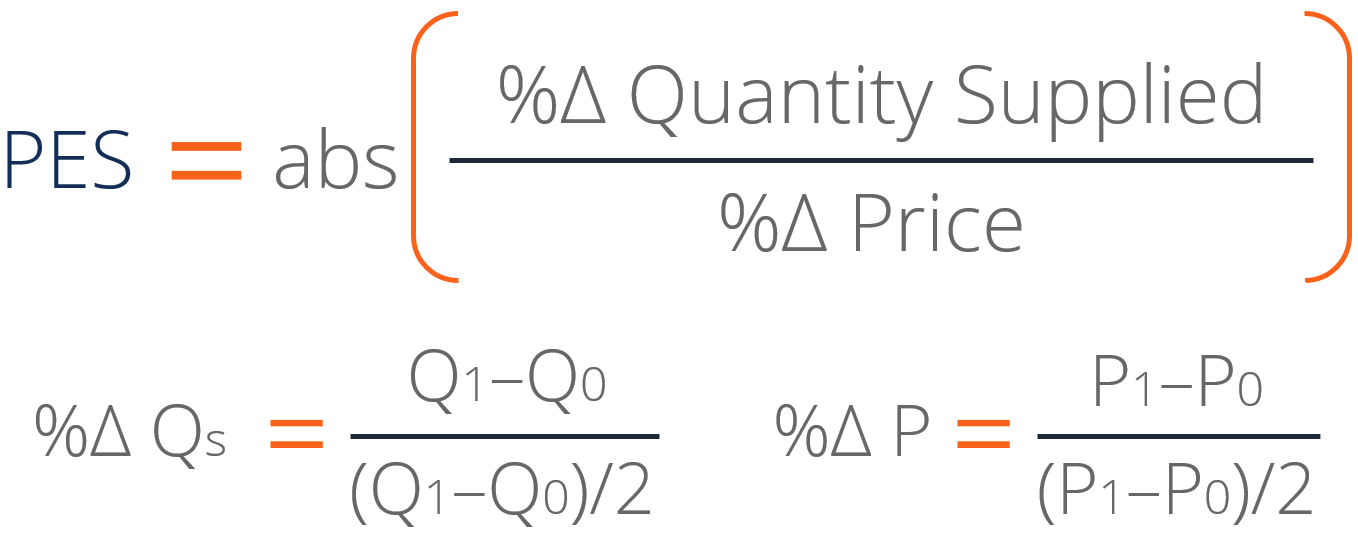For both demand and supply, the following categorizations hold true:However, we need to be mindful that supply slopes upwards while demand slopes downwards. Thus,

• Elastic PES would mean that increases in the price will lead to disproportionately large increases in quantity supplied.
• Inelastic PES would mean that increases in the price will lead to disproportionately small increases in quantity supplied.
• Unit elastic PES would mean that increases in the price will lead to proportionately equal increases in quantity supplied.

### More Resources

CFI offers the Financial Modeling & Valuation Analyst (FMVA)™ certification program for those looking to take their careers to the next level. To learn more about related topics, check out the following CFI resources:

• Gross Domestic Product (GDP)
• Market Cap to GDP Ratio (the Buffett Indicator)
• Fiscal Policy
• Debt vs Equity Financing

### Financial Analyst Certification

Become a certified Financial Modeling and Valuation Analyst (FMVA)® by completing CFI’s online financial modeling classes!